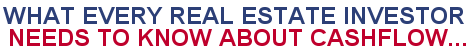Paperback: 261 pages Pub: McGraw-Hill ISBN: 0071422579 Available at:Amazon
Compound Interest Calculations
 PV %I N FV (per period) Calculate Future Value Calculate Present Value Calculate Periodic Rate Calculate Number of Periods Automatic Recalculation  Compound Interest Calculations The Four Calculators above will allow you to calculate the unknown variable when you have the three known variables of your compound interest problem. PV = Present Value %i = Periodic Interest Rate N = Number of Periods of the Loan FV = Future Value